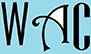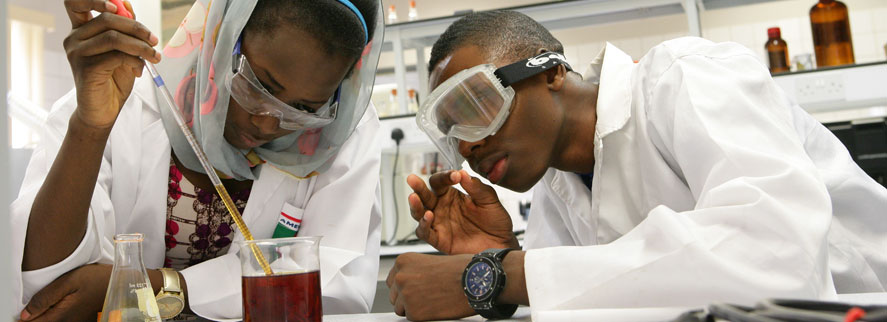## Algebra Question for Title 1 Schools: What Percent of a Number Question?

16) What percent of 90 is 27?

y% = y/100

of = x (multiply)

y/100 x 90 = 27

you have to make “y” subject of formula by moving “100” and “90” to the other side. When you go to the other side, addition becomes subtraction, multiplication becomes division, and vice versa.

y = (27 x 100)/ 90 (90 was multiplication while 100 was division at the other end. 27 never changed position)

y = 2700/90

y = 30%